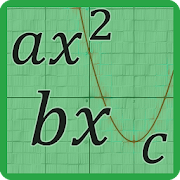# Quadratic Equation Solver with Steps and Graphs - Lumos Educational App Store4.71
Price -FREE
\$0

#### DESCRIPTION:

This is a smart application which solves quadratic equations or formulas and gives you the step-by-step solution. Unlike most of the other apps, this app is featured with both "Quadratic Formula" and "Completing the square" methods. â Capable of generating graphs for a given equation. â Both "Quadratic Formula" and "Completing the square" methods available. â Ability to save step-by-step solution as an image. â User friendly interface with Material design. â Decimal and fractional number inputs. â Decimal and fractional number output. â

#### OVERVIEW:

Quadratic Equation Solver with Steps and Graphs is a free educational mobile app By .It helps students in grades HS practice the following standards HSN.CN.C.9.

This page not only allows students and teachers download Quadratic Equation Solver with Steps and Graphs but also find engaging Sample Questions, Videos, Pins, Worksheets, Books related to the following topics.

1. HSN.CN.C.9 : Know the Fundamental Theorem of Algebra; show that it is true for quadratic polynomials..

HS

HSN.CN.C.9

#### ADDITIONAL INFORMATION:

Developer:

Software Version: 1.4.2

Category: Education

### RELATED APPS